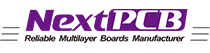### New Life with Your NEXTPCB!

Turnkey PCB manufacturing & assembly services.

## Right-angle alignment will have any impact in PCB layout

writer: G December 26, 2017

Right-angle alignment of the signal impact is mainly reflected in three aspects: First, the corner can be equivalent to the capacitive load on the transmission line, slow down the rise time; Second, the impedance discontinuity will cause the signal reflection; Third, the parasitic capacitance at the right angle of the transmission line can be calculated from the following empirical formula: C = 61W (Er) 1/2 / Z0. In the above equation, C refers to the equivalent capacitance of the corner (in pF), W refers to the width of the trace (unit: inch), εr refers to the dielectric constant of the medium, and Z0 is the characteristic impedance of the transmission line.

As the line width of the rectangular alignment increases, the impedance there will be reduced, so there will be a certain signal reflection phenomenon. The equivalent impedance after the line width increase can be calculated according to the impedance calculation formula, and then the reflection coefficient is calculated according to empirical formula: ρ = (Zs-Z0) / (Zs + Z0), the impedance change caused by the normal right-angle alignment is between 7% and 20%, so the maximum reflection coefficient is about 0.1.

• PCB
• SMT
• Stencil
 Dimensions:(mm) × Quantity: 5 10 15 20 25 30 40 50 75 100 120 150 200 250 300 350 400 450 500 600 700 800 900 1000 1500 2000 2500 3000 3500 4000 4500 5000 5500 6000 6500 7000 7500 8000 8500 9000 Layers: 1 2 4 6 8 Thickness: 0.6 mm 0.8 mm 1.0 mm 1.2 mm 1.6 mm 2.0 mm 2.5 mm Quote now